Search IntMath
Close

450+ Math Lessons written by Math Professors and Teachers

5 Million+ Students Helped Each Year

1200+ Articles Written by Math Educators and Enthusiasts

Simplifying and Teaching Math for Over 23 Years

# Context Free math-based art

By Murray Bourne, 31 Jul 2014

## Context Free

Context Free is software that you can use to generate fascinating art work. You give instructions using code called a "grammar". It's quite a neat way to bring together math, art and programming, which are key STEM skills.

The Context Free Art site provides downloads of the (free) software (Mac, Windows and Linux), and also a gallery of around 1000 works of art generated using the program.

Everything is released using a Creative Commons non-commercial license, so you can use the images in your own work. You can modify other people's code to create your own materpieces (but give credit to the original, of course).

Here are some of the output images from the gallery: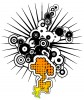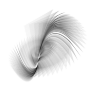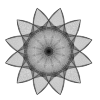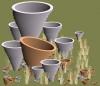## Math involved

Drawing these images involves some interesting math topics, including:

• Trigonometry
• Logarithms
• Matrices
• Vectors
• Geometry
• Number theory
• Complex numbers

## Example image

This one is called the Lorenz Attractor, by thijs: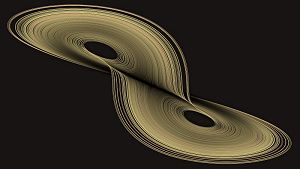To give you an idea what is involved, this is the code thijs wrote to produce the above shape:

startshape Lorenz_Attractor //1600*900

CF::Background = [b -0.9 sat 0.2]
CF::Size=[s 60 33.75]
CF::ColorDepth = 16
CF::MinimumSize= 0.3
CF::Impure = 1

A=10 B=8/3 C=28 d=0.002
vector3 D(vector3 V)
=V+d*( A*(V-V) )
,V+d*( V*(C-V)-V )
,V+d*( V*V-B*V )

vector3 M(vector3 V1,vector3 V2)
=(V1+V2)/2
,(V1+V2)/2
,(V1+V2)/2

//------------------------------------
shape Lorenz_Attractor
{ V0=(-12.5, -17.4, 26)
Next(0,V0)[r 100]
}

shape Next (i,vector3 Vi)
{ if (i<320000)
{ Vn=M(Vi,D(D(Vi)))
Plot (i,Vi,Vn) []
Next (i+1 ,Vn) []
} }

shape Plot(i,vector3 V0,vector3 V1)
{ P=V0[0,2] Q=V1[0,2] Z=V0
H=45+15*sin(i/3)
Line(0.05,P,Q) [z Z b 1 h H sat .4]
Line(0.15,P,Q) [z Z b -1 a -.950]
Line(1.50,P,Q) [z Z b -1 a -.999]
}

path Line(S,vector2 P,vector2 Q)
{ MOVETO(P) LINETO(Q)
STROKE(S,CF::RoundCap)[] 

Be the first to comment below.

### Comment Preview

HTML: You can use simple tags like <b>, <a href="...">, etc.

To enter math, you can can either:

1. Use simple calculator-like input in the following format (surround your math in backticks, or qq on tablet or phone):
a^2 = sqrt(b^2 + c^2)
(See more on ASCIIMath syntax); or
2. Use simple LaTeX in the following format. Surround your math with $$ and $$.
$$\int g dx = \sqrt{\frac{a}{b}}$$
(This is standard simple LaTeX.)

NOTE: You can mix both types of math entry in your comment.